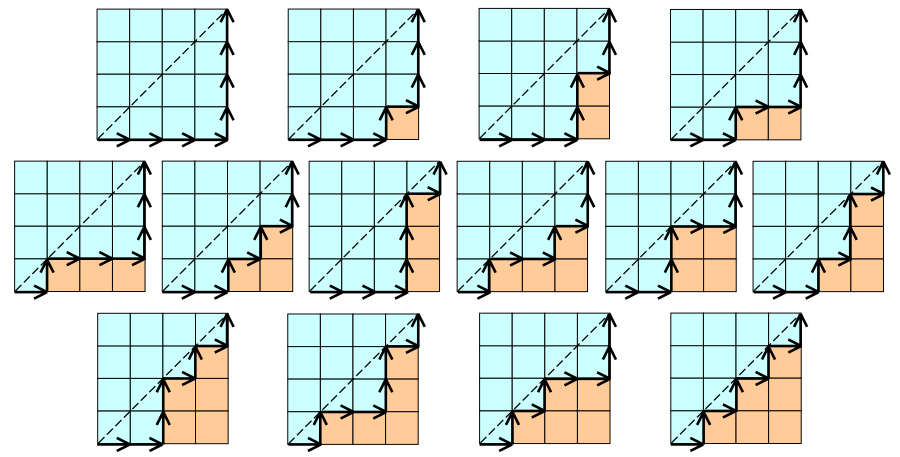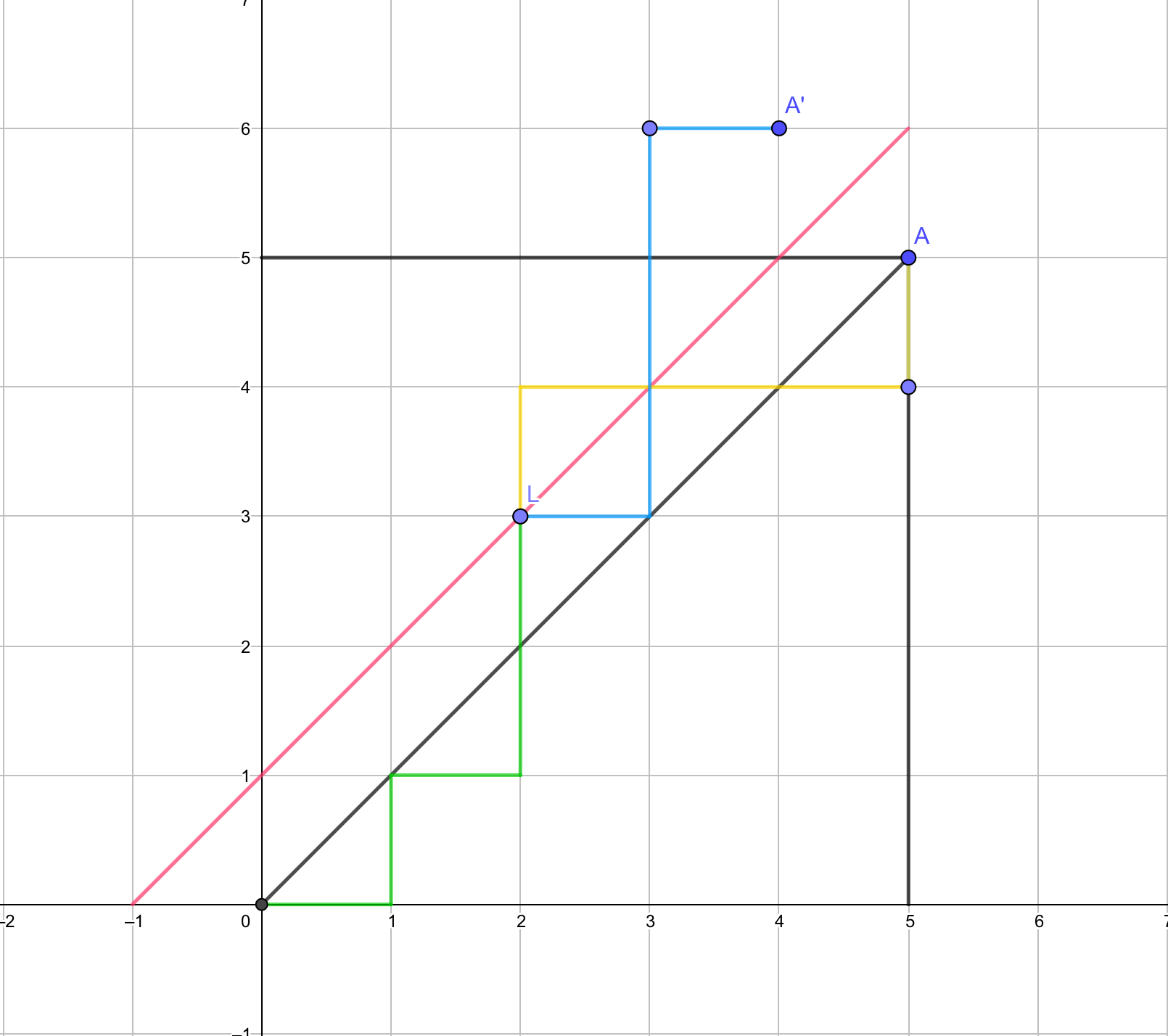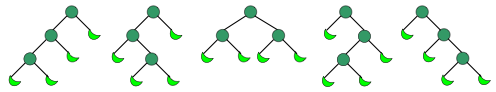# 卡特兰数

## 卡特兰数的意义

$$C_n$$表示所有在n × n格点中不越过对角线的单调路径的个数。

## 通项公式

$C_{n}=\frac{1}{n+1}\left(\begin{array}{c}{2 n} \\ {n}\end{array}\right)=\frac{(2 n) !}{(n+1) ! n !}$

$\displaystyle C_n=\binom{2n}{n}-\binom{2n}{n+1}\quad \operatorname{for} n\ge1$

## 卡特兰数通项公式的证明$C_{n}=\left(\begin{array}{c}{2 n} \\ {n}\end{array}\right)-\left(\begin{array}{c}{2 n} \\ {n+1}\end{array}\right) \quad \text { for } n \geq 1$

## 应用

• $$C_n$$表示长度2n的dyck word的个数。Dyck word是一个有n个X和n个Y组成的字串，且所有的前缀字串皆满足X的个数大于等于Y的个数。以下为长度为6的dyck words: XXXYYY XYXXYY XYXYXY XXYYXY XXYXYY。（这个性质在ZROI1151用到了）
• 将上例的X换成左括号，Y换成右括号，$$C_n$$表示所有包含n组括号的合法运算式的个数。
• $$C_n$$表示有n个节点组成不同构的二叉树方案数。下图中，n等于3，圆形表示节点，月牙形表示什么都没有。（不同构二叉树是指：对于两颗二叉树每个节点，他们的左儿子和右儿子完全相等）
• $$C_n$$表示有2n+1个节点组成不同构"合并果子树"（full binary tree）的方案数。下图中，n等于3，圆形表示内部节点，月牙形表示外部节点。本质同上。• $$C_n$$表示通过连结顶点而将n + 2边的凸多边形分成三角形的方法个数。
• $$C_n$$表示集合$$\{1,...,n\}$$依次入栈后合法的出栈顺序方案数。

## 参考资料posted @ 2019-10-20 08:55  GavinZheng  阅读(2292)  评论(0编辑  收藏  举报